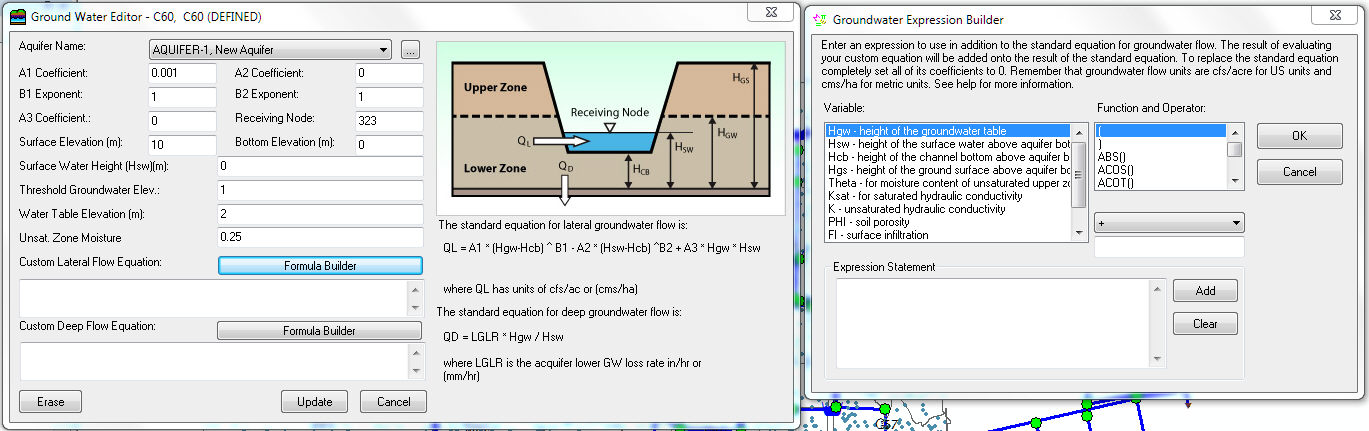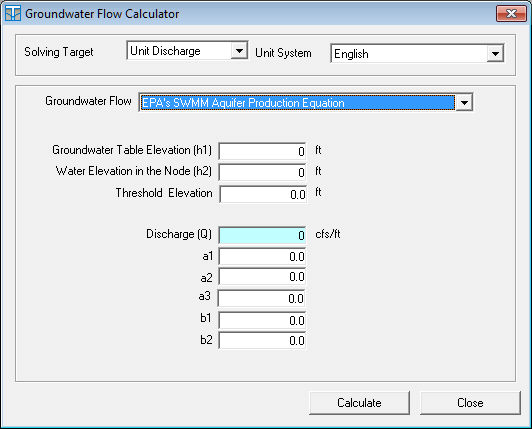#INFOSWMM

# Groundwater Equation Editor for #InfoSWMM and #H2OMap SWMM

The GroundwaterEquation Editor is used to supply a custom equation for computing groundwater flow between the saturated sub-surface zone of a subcatchment and either a node in the conveyance network (lateral flow) or to a deeper groundwater aquifer (deep flow). It is invoked from the GroundwaterFlow Editor form.For lateral groundwater flow the result of evaluating the custom equation will be added onto the result of the standard equation. To replace the standard equation completely set all of its coefficients to 0. Remember that groundwater flow units are cfs/acre for US units and cms/ha for metric units.

The following symbols can be used in the equation:

 Hgw (height of the groundwater table) Hsw (height of the surface water) Hcb (height of the channel bottom) Hgs (height of the ground surface) Phi (porosity of the subsurface soil) Theta (moisture content of the upper unsaturated zone) Ks (saturated hydraulic conductivity in inches/hr or mm/hr) K (hydraulic conductivity at the current moisture content in inches/hr or mm/hr) Fi (infiltration rate from the ground surface in inches/hr or mm/hr) Fu (percolation rate from the upper unsaturated zone in inches/hr or mm/hr) A (subcatchment area in acres or hectares)

where all heights are relative to the aquifer's bottom elevation in feet (or meters).

The STEP function can be used to have flow only when the groundwater level is above a certain threshold. For example, the expression:

0.001 * (Hgw - 5) * STEP(Hgw - 5)

would generate flow only when Hgw was above 5. See the Treatment Editor topic for a list of additional math functions that can be used in a groundwater flow expression.

# EPA SWMM’s Aquifer Production Equation from the Innovyze H2OCalc Help File

The dialog box for EPA SWMM’s aquifer production equation is shown below.

Input for EPA SWMM’s aquifer production equation:

· Unit System – English or SI unit.

· Solving Target – Unit discharge.

· Groundwater Flow – Select EPA SWMM’s aquifer production equation.

· Hydraulic Conductivity – A proportionality constant that describes the ease with which water can move through pore spaces of the aquifer. It is an output if selected as a solving target.

· Groundwater Table Elevation – Elevation of the groundwater table.

· Water Elevation in the Node – Elevation of surface water at the receiving node.

· Threshold Elevation – Threshold groundwater table elevation or elevation of node invert.

· a1, a2, b1, b2, and a3 – coefficients that appear in the following equation that computes groundwater flow as a function of groundwater and surface water heads.Output for EPA SWMM’s aquifer production equation:

· Discharge – Flow rate per unit width of the aquifer.